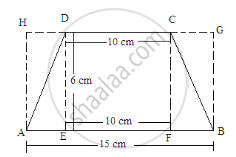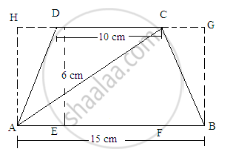Share

# Find the Area of a Trapezium Whose Parallel Sides of Lengths 10 Cm and 15 Cm Are at a Distance of 6 Cm from Each Other. Calculate this Area as the - Mathematics

Course
ConceptArea of Trapezium

#### Question

Find the area of a trapezium whose parallel sides of lengths 10 cm and 15 cm are at a distance of 6 cm from each other. Calculate this area as the difference of the area of a rectangle and the sum of the areas of two triangles.

#### Solution

Given:
Length of the parallel sides of a trapezium are 10 cm and 15 cm.
The distance between them is 6 cm.
Let us extend the smaller side and then draw perpendiculars from the ends of both sides.In this case, the figure will look as follows:Area of trapezium ABCD=(Area of rectangle ABGH)-[(Area of triangle AHD)+(Area of triangle BGC)]
$= (15 \times 6) - [(\frac{1}{2} \times DH \times 6) + (\frac{1}{2} \times GC \times 6)]$
$= 90 - [3\times DH + 3 \times GC]$
= 90 - 3[DH+GC]
Here, HD+DC+CG=15 cm
DC=10 cm
HD+10+CG=15
HD+GC=15-10=5 cm
Putting this value in the above equation:
${\text{ Area of the trapezium }=90-3(5)=90-15=75 cm}^2$
Is there an error in this question or solution?

#### APPEARS IN

RD Sharma Solution for Mathematics for Class 8 by R D Sharma (2019-2020 Session) (2017 to Current)
Chapter 20: Mensuration - I (Area of a Trapezium and a Polygon)
Ex. 20.2 | Q: 7.2 | Page no. 22

#### Video TutorialsVIEW ALL 

Solution Find the Area of a Trapezium Whose Parallel Sides of Lengths 10 Cm and 15 Cm Are at a Distance of 6 Cm from Each Other. Calculate this Area as the Concept: Area of Trapezium.
S